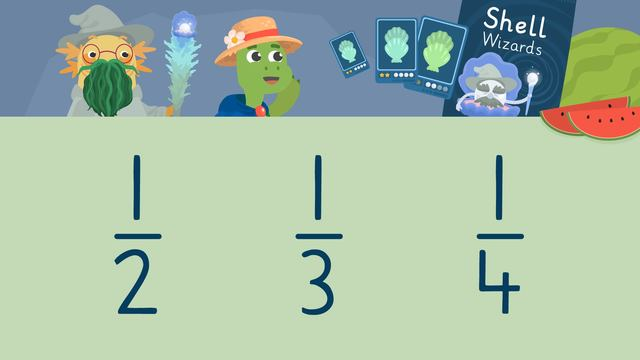# What are fractions?Rate this video

Ø 5.0 / 1 ratings

The authorTeam Digital
What are fractions?
CCSS.MATH.CONTENT.3.NF.A.1

## Information about the videoWhat are fractions?

### In This Video

Axel and Tank are preparing for game night, but on their shopping list are fractional items that they need. They wish to understand ‘what are fractions?’ so they can successfully buy everything needed for game night.

### What Are Fractions in Math?

What are fractions used for? Fractions in math are used to represent equal parts of a whole or equal parts of a group. Below are examples of equal parts of a whole and equal parts of a group.### What are Two Big Ideas in the Learning of Fractions?

The first big idea is that fractions are represented with a numerator, the top number, and the denominator, the bottom number. These are separated by a fraction bar.The second big idea is that fractions represent an equal portion of a whole or a group. For example, they could represent one-half of a whole watermelon.Another example is that fractions could also represent an equal part of a collection, or group, of cards.### What are Fractions Summary

Fractions represent an equal part of a whole or group. They have a numerator, a denominator, with a fraction bar that separates them. Below you will find worksheets and practice problems.

### TranscriptWhat are fractions?

Axel and Tank are running errands for game night! "Axel, look at this on our list, what's that?" "Oh, that Tank, is called a fraction!" Let's help answer Tanks question what are fractions? You may have seen fractions before, like these! So, what exactly are fractions? Fractions are equal parts of a whole or a group! Fractions are used when we talk about parts of a whole, or parts of a group! To show this, fractions are written in two parts. The top number, called a numerator, represents the equal parts of the whole you have. The bottom number, called a denominator, represents the total equal parts of the whole or group. They are divided by a line called a fraction bar. For example, Axel and Tanks grocery list says they need one half of a whole watermelon for game night. In this situation, we are talking about a part of a whole. The entire watermelon represents the whole. Since Axel and Tank want one half, divide the watermelon into two equal parts because the denominator tells us the whole has TWO parts. The numerator is one, so we take one part of the two parts of watermelon! Now they have their one half of a watermelon, what about the other item they have purchased? They need one-fourth of the twelve cards they purchased to complete their set for game night. First, let's open the card packet and get all twelve cards out. Because there is more than one whole this time, they want a fraction of a group! This time, we would identify the denominator, which is four. Then share all twelve cards into four equal groups, like this. Each group ends up with three cards in it. Now look at the numerator, which asks for one part of the four groups. They take one of these groups, which has three cards in it. One quarter of a packet of twelve cards is three cards! While Axel and Tank head home for game night, let's review! Remember, fractions represent equals part of a whole or group. Fractions consist of a numerator and a denominator, which are separated by a fraction bar. Fractions can represent parts of a whole or they can represent parts of a group. "And with this card, I claim victory!" "mwahahaha!" "No! You have defeated me! Oh well! Same time again next week?"